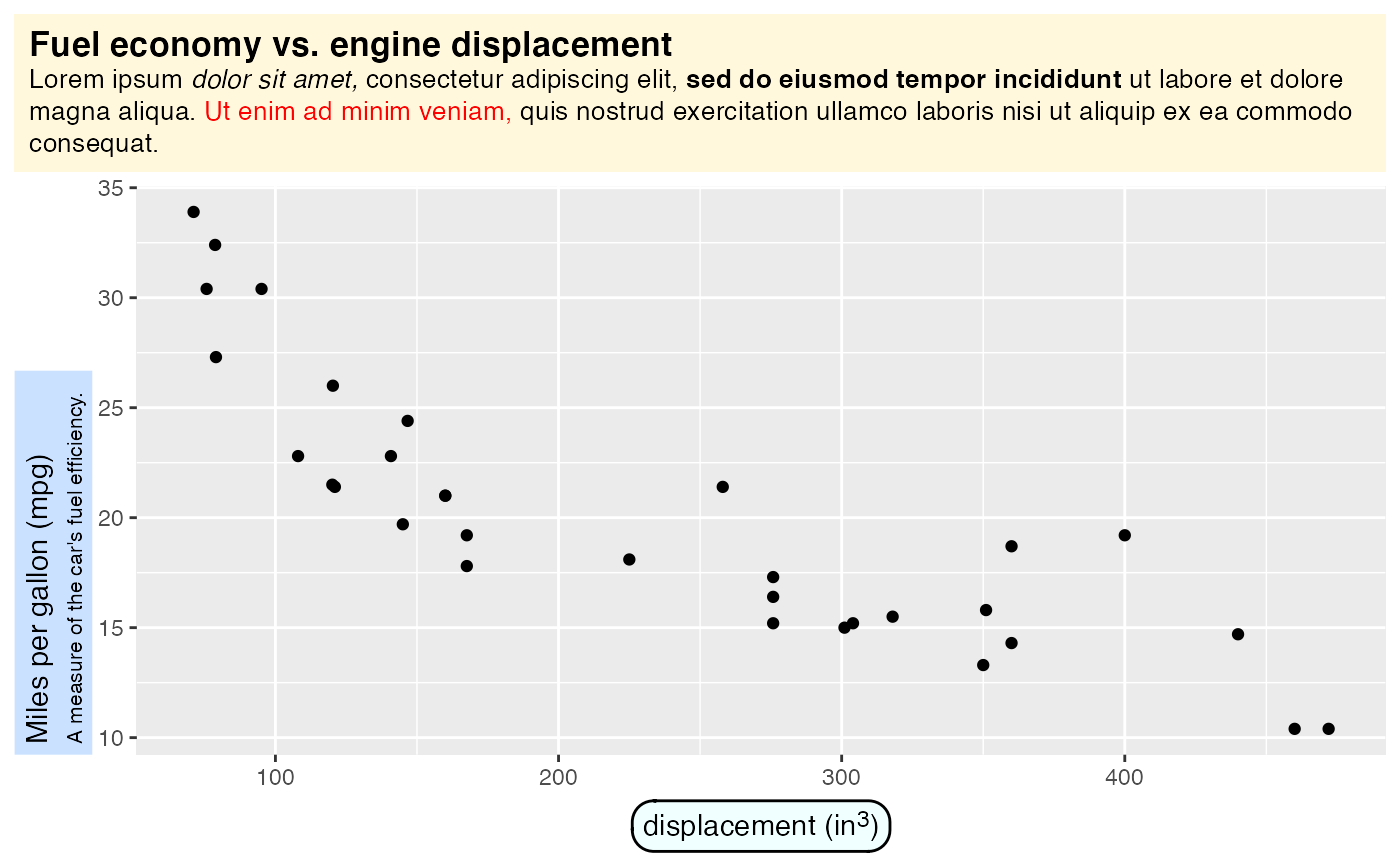The theme elements element_textbox() and element_textbox_simple() enable Markdown text in a box, with word wrap. Both functions implement exactly the same functionality; they only differ in the default values for the various element values. element_textbox() sets all values that are not specified to NULL, as is the usual practice in ggplot2 themes. These missing values are usually completed by inheritance from parent theme elements. By contrast, element_textbox_simple() provides meaningful default values for many of the values that are not usually defined in ggplot2 themes. This makes it simpler to use a textbox element in the context of an existing theme.

element_textbox(
family = NULL,
face = NULL,
size = NULL,
colour = NULL,
fill = NULL,
box.colour = NULL,
linetype = NULL,
linewidth = NULL,
hjust = NULL,
vjust = NULL,
halign = NULL,
valign = NULL,
lineheight = NULL,
margin = NULL,
width = NULL,
height = NULL,
minwidth = NULL,
maxwidth = NULL,
minheight = NULL,
maxheight = NULL,
r = NULL,
orientation = NULL,
color = NULL,
box.color = NULL,
debug = FALSE,
inherit.blank = FALSE
)

element_textbox_simple(
family = NULL,
face = NULL,
size = NULL,
colour = NULL,
fill = NA,
box.colour = NULL,
linetype = 0,
linewidth = 0.5,
hjust = 0.5,
vjust = 0.5,
halign = 0,
valign = 1,
lineheight = 1.2,
margin = ggplot2::margin(0, 0, 0, 0),
padding = ggplot2::margin(0, 0, 0, 0),
width = grid::unit(1, "npc"),
height = NULL,
minwidth = NULL,
maxwidth = NULL,
minheight = NULL,
maxheight = NULL,
r = grid::unit(0, "pt"),
orientation = "upright",
color = NULL,
box.color = NULL,
debug = FALSE,
inherit.blank = FALSE
)

## Arguments

family Font family Font face Font size (in pt) Text color Fill color of the enclosing box Line color of the enclosing box (if different from the text color) Line type of the enclosing box (like lty in base R) Line width of the enclosing box (measured in mm, just like size in ggplot2::element_line()). Horizontal justification Vertical justification Horizontal justification Vertical justification Line height, in multiples of the font size Padding and margins around the text box. See gridtext::textbox_grob() for details. Unit objects specifying the width and height of the textbox, as in gridtext::textbox_grob(). Min and max values for width and height. Set to NULL to impose neither a minimum nor a maximum. Unit value specifying the corner radius of the box Orientation of the text box. See gridtext::textbox_grob() for details. Not implemented. See ggplot2::margin() for details.

## Value

A ggplot2 theme element that can be used inside a ggplot2::theme() call.

gridtext::textbox_grob(), element_markdown(), geom_textbox()

## Examples

library(ggplot2)

ggplot(mtcars, aes(disp, mpg)) +
geom_point() +
labs(
title =
"<b><span style = 'font-size:13pt'>Fuel economy vs. engine displacement</span></b><br>
Lorem ipsum *dolor sit amet,* consectetur adipiscing elit, **sed do eiusmod tempor
incididunt** ut labore et dolore magna aliqua. <span style = 'color:red;'>Ut enim ad
minim veniam,</span> quis nostrud exercitation ullamco laboris nisi ut aliquip ex ea
commodo consequat.",
x = "displacement (in<sup>3</sup>)",
y = "Miles per gallon (mpg)<br><span style = 'font-size:8pt'>A measure of
the car's fuel efficiency.</span>"
) +
theme(
plot.title.position = "plot",
plot.title = element_textbox_simple(
size = 10,
padding = margin(5.5, 5.5, 5.5, 5.5),
margin = margin(0, 0, 5.5, 0),
fill = "cornsilk"
),
axis.title.x = element_textbox_simple(
width = NULL,
padding = margin(4, 4, 4, 4),
margin = margin(4, 0, 0, 0),
linetype = 1,
r = grid::unit(8, "pt"),
fill = "azure1"
),
axis.title.y = element_textbox_simple(
hjust = 0,
orientation = "left-rotated",
minwidth = unit(1, "in"),
maxwidth = unit(2, "in"),
padding = margin(4, 4, 2, 4),
margin = margin(0, 0, 2, 0),
fill = "lightsteelblue1"
)
)